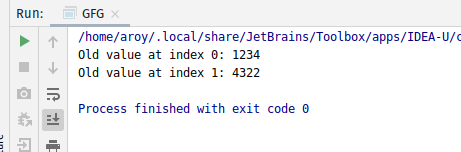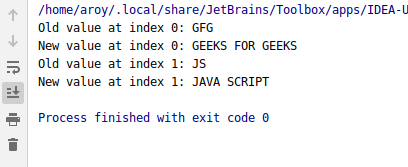# AtomicReferenceArray compareAndExchange() method in Java with Examples

• Last Updated : 03 Jan, 2020

The compareAndExchange() method of a AtomicReferenceArray class is used to atomically sets the value of index i of AtomicReferenceArray to newValue, if the current value at index i of AtomicReferenceArray object which is referred to as the witness value is equal to the expectedValue. This method will return the witness value, which will be the same as the expected value. This method handles the operation with memory semantics of reading as if the variable was declared volatile.

Syntax:

```public final boolean
compareAndExchange(
int i, E expectedValue, E newValue)
```

Parameters: This method accepts:

• i which is an index of AtomicReferenceArray to perform the operation,
• expectedValue which is the expected value and
• newValue which is the new value to set.

Return value: This method returns the witness value, which will be the same as the expected value if successful.

Below programs illustrate the compareAndExchange() method:
Program 1:

 `// Java program to demonstrate``// compareAndExchange() method`` ` `import` `java.util.concurrent.atomic.*;`` ` `public` `class` `GFG {``    ``public` `static` `void` `main(String[] args)``    ``{``        ``// create an atomic reference object.``        ``AtomicReferenceArray ref``            ``= ``new` `AtomicReferenceArray(``3``);`` ` `        ``// set some value``        ``ref.set(``0``, ``1234``);``        ``ref.set(``1``, ``4322``);`` ` `        ``// apply compareAndExchange()``        ``int` `oldV1``            ``= ref.compareAndExchange(``0``, ``1234``, ``8913``);``        ``int` `oldV2``            ``= ref.compareAndExchange(``1``, ``4322``, ``6543``);`` ` `        ``// print``        ``System.out.println(``"Old value at index 0: "``                           ``+ oldV1);``        ``System.out.println(``"Old value at index 1: "``                           ``+ oldV2);``    ``}``}`

Output:Program 2:

 `// Java program to demonstrate``// compareAndExchange() method`` ` `import` `java.util.concurrent.atomic.*;`` ` `public` `class` `GFG {``    ``public` `static` `void` `main(String[] args)``    ``{``        ``// create an atomic reference object.``        ``AtomicReferenceArray ref``            ``= ``new` `AtomicReferenceArray(``3``);`` ` `        ``// set some value``        ``ref.set(``0``, ``"GFG"``);``        ``ref.set(``1``, ``"JS"``);`` ` `        ``// apply compareAndExchange()``        ``String oldV1``            ``= ref.compareAndExchange(``                ``0``, ``"GFG"``,``                ``"GEEKS FOR GEEKS"``);``        ``String oldV2``            ``= ref.compareAndExchange(``                ``1``, ``"JS"``,``                ``"JAVA SCRIPT"``);`` ` `        ``// print``        ``System.out.println(``"Old value at index 0: "``                           ``+ oldV1);``        ``System.out.println(``"New value at index 0: "``                           ``+ ref.get(``0``));``        ``System.out.println(``"Old value at index 1: "``                           ``+ oldV2);``        ``System.out.println(``"New value at index 1: "``                           ``+ ref.get(``1``));``    ``}``}`

Output:My Personal Notes arrow_drop_up